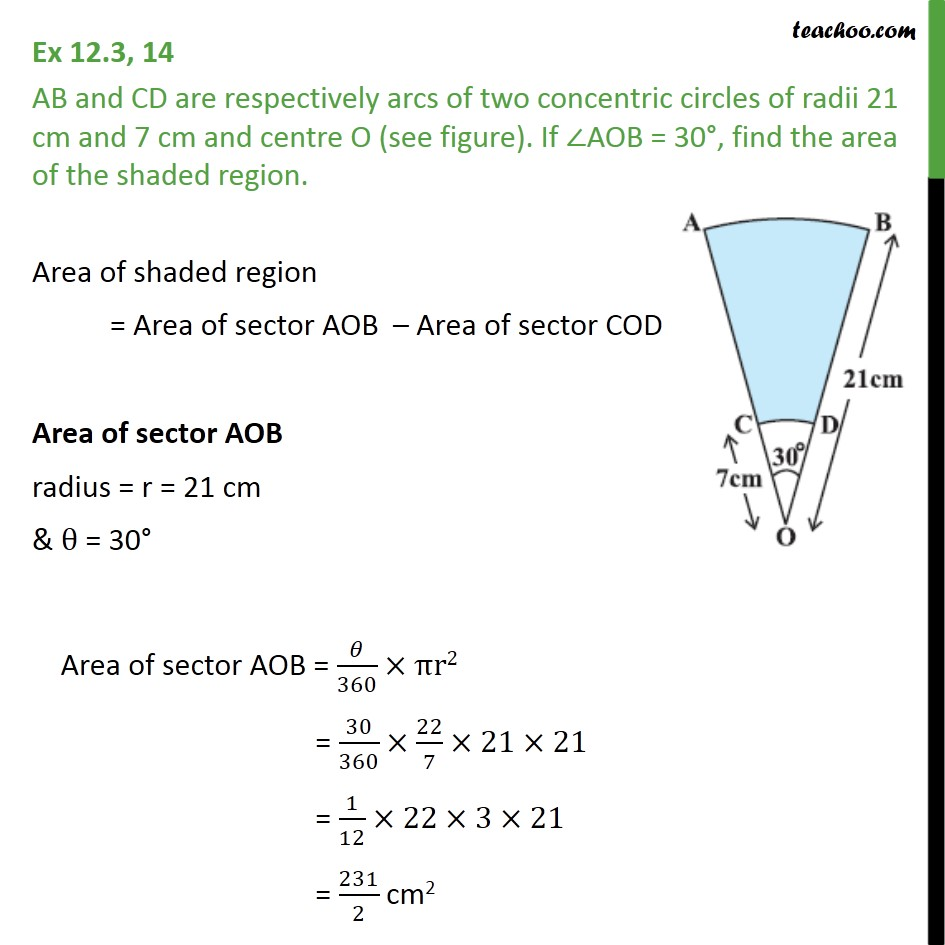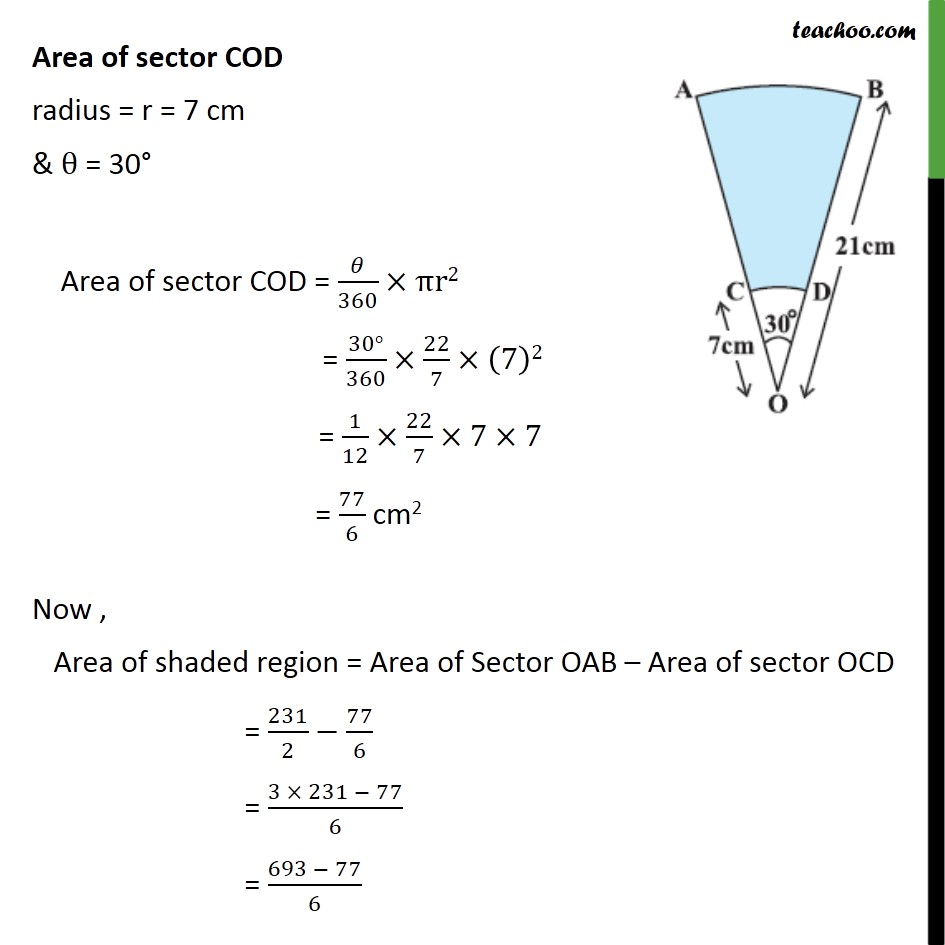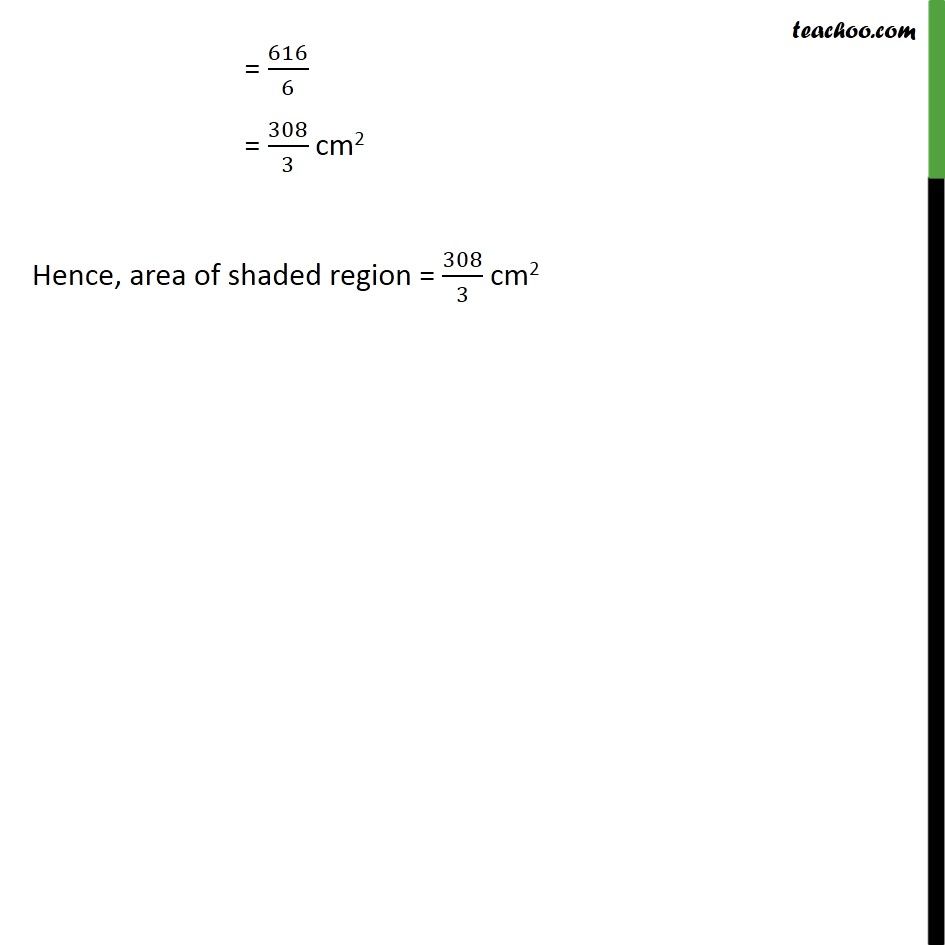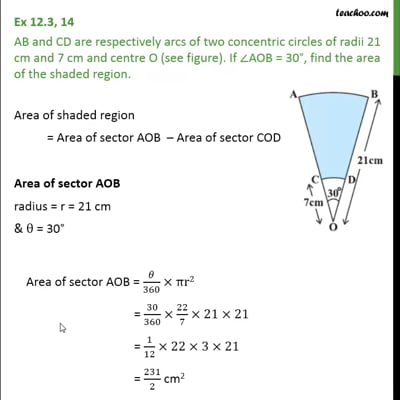Area of combination of figures : sector based

Chapter 12 Class 10 Areas related to Circles
Concept wiseThis video is only available for Teachoo black users

Solve all your doubts with Teachoo Black (new monthly pack available now!)

### Transcript

Ex 12.3, 14 AB and CD are respectively arcs of two concentric circles of radii 21 cm and 7 cm and centre O (see figure). If AOB = 30 , find the area of the shaded region. Area of shaded region = Area of sector AOB Area of sector COD Area of sector AOB radius = r = 21 cm & = 30 Area of sector AOB = /360 r2 = 30/360 22/7 21 21 = 1/12 22 3 21 = 231/2 cm2 Area of sector COD radius = r = 7 cm & = 30 Area of sector COD = /360 r2 = (30 )/360 22/7 (7)2 = 1/12 22/7 7 7 = 77/6 cm2 Now , Area of shaded region = Area of Sector OAB Area of sector OCD = 231/2 77/6 = (3 231 77)/6 = (693 77)/6 = 616/6 = 308/3 cm2 Hence, area of shaded region = 308/3 cm2